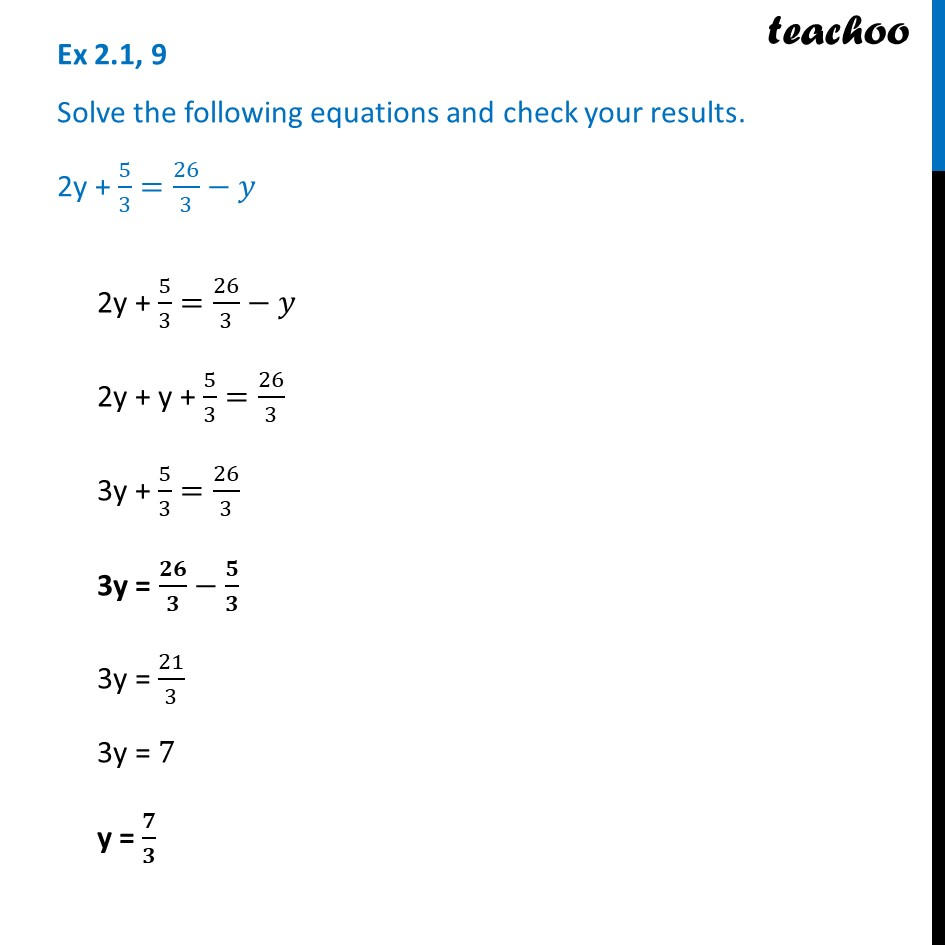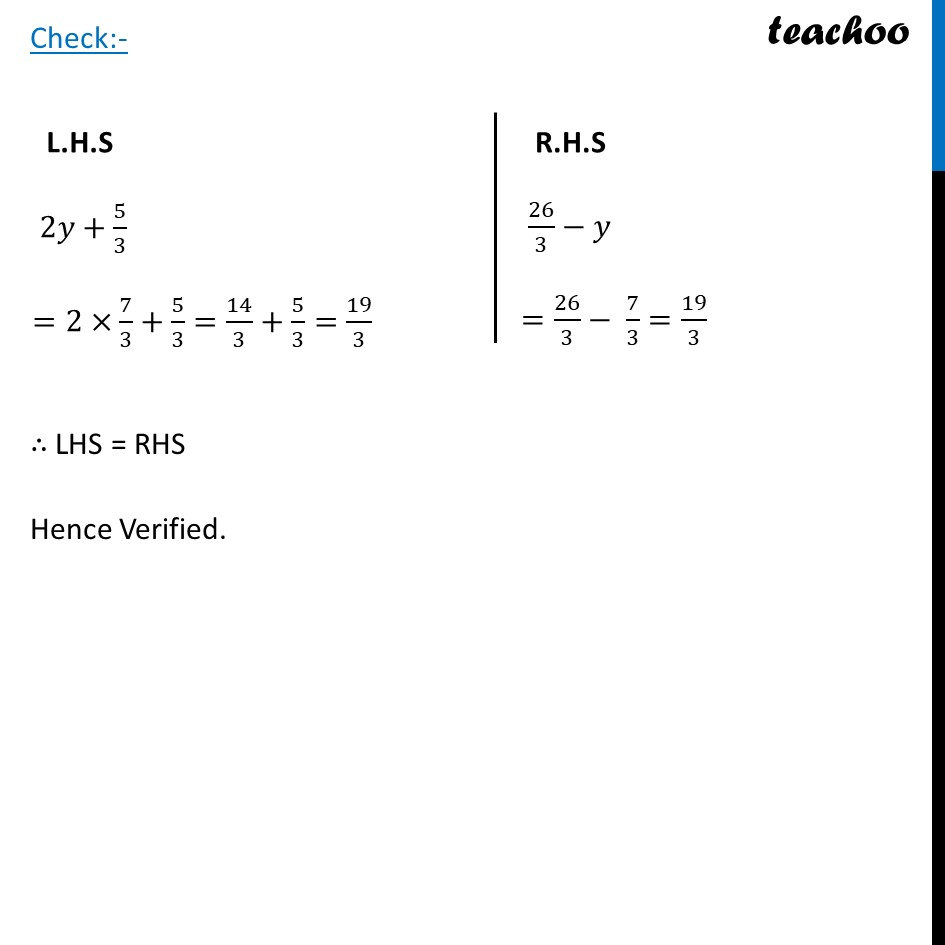Ex 2.1

Chapter 2 Class 8 Linear Equations in One Variable
Serial order wiseLearn in your speed, with individual attention - Teachoo Maths 1-on-1 Class

### Transcript

Ex 2.1, 9 Solve the following equations and check your results. 2y + 5/3=26/3−𝑦2y + 5/3=26/3−𝑦 2y + y + 5/3=26/3 3y + 5/3=26/3 3y = 𝟐𝟔/𝟑−𝟓/𝟑 3y = 21/3 3y = 7 y = 𝟕/𝟑 L.H.S 2𝑦+5/3 =2×7/3+5/3=14/3+5/3=19/3 R.H.S 26/3−𝑦 =26/3− 7/3=19/3 Check:- ∴ LHS = RHS Hence Verified.